# LogOptimize: what’s the meaning of this word?

Logoptimization is a new word (obtained by contracting “logic” and “optimization) that stands for “optimization with logic variables”. A logic variable, also called a binary variable, can only take one of two possible values like, e.g., 0 and 1, or -1 and 1, or 5 and 44, or FALSE and TRUE, or RED and BLUE, and so on. We assume here for simplicity, but with no loss of generality, that these two values are 0 and 1.

A logoptimization problem is the following: a function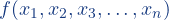on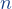logic variable is given. For each assignment of the values 0 and 1 to the variables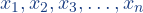the corresponding value of the function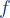is a real number. Then the problem is to find an assignment for which the value ofis maximal (or minimal) and to provide mathematical evidence that no other assignment performs better. This evidence is called an optimality certificate.

An example
For example, suppose that the function f  to be maximized is the polynomial in the three variables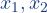, and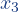: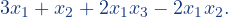The following table shows the value of the function for all possible assignments of its variables: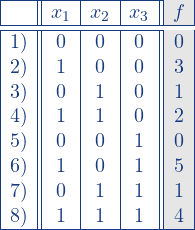Evidently, the best solution corresponds to the sixth assignment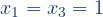,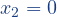.

How difficult is it to solve a logoptimization problem?
For an arbitrary function onvariables, the number of possible distinct assignments is finite, as there are only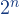of them. Therefore, finding the solution to the problem is conceptually rather simple, since it amounts to computing the function for each possible pattern ofsymbols in the set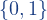and to taking the pattern that corresponds to the maximal (minimal) value attained by the function. This simple solution strategy is called complete enumeration or optimization by brute force. However, since the function, that gives the number of function evaluations, grows very rapidly asincreases (recall the story of Sissa ben Dahir and the grains of wheat on the chessboard), complete enumeration becomes computationally expensive, or even computationally unfeasible, already for moderate values of the parameter.

Yes, but computers are getting more and more powerful …
Unfortunately, technological advances in the computer industry cannot help much. To make it more clear, let us assume that we can get access to an ideal parallel computer with as many computing units as the atoms in the universe (estimated to be roughly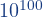). Let us also assume that each unit is able to perform a billion function evaluations per second. Well, such a colossal technological jewel would take more than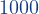years to solve a logoptimization problem by brute force when the value of the parameteris only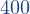.

And what if we want to keep our feet on the ground and go down to a more realistic scenario?
Let us say that the computer on our desk can execute a billion function evaluations per second and that we can wait only twenty minutes to get the result. Then we cannot cope with problems whereis larger than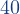.

Is there a need for methods faster than brute force?
There are uncountable real problems in Computer Science, Electrical Engineering, Management Science, Decision Science, Data Mining, Machine Learning and in many other fields, that can be formulated as logoptimization problems with several hundred, or even thousand, variables. Therefore, a great challenge for scientists working in the area of Mathematical Optimization is to devise effective methods that are able to solve problems of these sizes in a reasonable amount of computation time.

The LogOptimize solver
WE.LOGOPTIMIZE.IT is the web site of a virtual laboratory where an international group of researchers develops methods, algorithms, and software for the solution (with optimality certificate) of logoptimization problems. The main purpose of the site is to provide the interested user with friendly access to a server dedicated to the solution of these problems.
You can browse within the web site to get more insights, references, documentation, and other material, and to find out how to use the server to solve your logoptimization problems.

Enjoy the navigation and … give us your problem … we logoptimize it !!